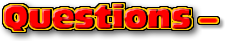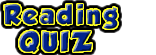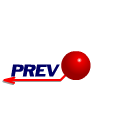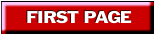ChaptersThis text is meant to accompany class discussions. It is not everything there is to know about the basics of electricity. It is meant as a  prep for class. More detailed notes and examples are given in the class notes, presentations, and demonstrations (click here.)Click for the questions that go with this reading
Magnitude and Direction

A component is the a smaller piece of a bigger body. These smaller pieces add up to create a resultant vector. For example, in general terms your car has a body, power source, braking system, and an electrical system. These four items add up to equal your car. The human body has 206 bones (if the sternum is considered one bone instead of three.) These 206 bones add up to be the skeleton. All these smaller pieces ad up to give you something bigger.

When discussing components of vectors, to "add" literally means to perform a mathematical summation operation on them. Below is the vector "R" drawn on a grid. The blue vectors are 2 of the components of the red vector.Note how the blue vectors show the process of addition. The tails meet at the left; the blue vectors connect tip-to-tail, and the tips (red and blue) meet. An infinite number of vectors can be draw such that the red vector is the sum of them. Below is an example of three vectors that add up to give the same resultant.All of these green vectors are components of the red vector, R. They all connect tip-to-tail to add up to equal R's magnitude and direction.

The two components of interest are the one's that combine to make a right triangle. The right triangle is easy to apply trigonometric functions to. On this grid, the components' magnitudes can be found by counting the blocks.To find the magnitude, use Pythagoreans theorem,Example calculations...It is possible to be given something like the problem below.

 Example

Notice a couple of things.

1. The vectors are moved such that the connect tip-to-tail.
2. The resultant starts as the tail and the ends at the tip.
3. The numbers all have units. The answer has the same units as the components. (This is may be different from your math classes where the units are seldom a concern.)

To find the direction, use right triangle trigonometry. Click here if you need a quick review of trigonometry.There are three functions that are needed for right triangle trig.There relationship are often remembered by the phrase, "SOH CAH TOA". Pronounced "so cah toe-ah." This stands for Sin is Opposite over Hypotenuse, Cosine is Adjacent over Hypotenuse, Tangent is Opposite over Adjacent. In the case of the picture above, the side opposite to the angle is known and the side adjacent to angle is known. The tang net function relates these two sides.Make sure you look at the diagram to see where the numbers come from.

 Example

But the angle is not enough. There needs to be more to describe where the angle of zero degrees is located. Below is an example of a problem.The vectors associated are both 27 degrees. Yet each vector is clearly pointing in different directions. There are two ways ways to describe the difference in these two vectors. Method one is to add the angle to a drawing OR to use a polar coordinate system. A vector pointing directly to the right is at zero degrees. All other angles are measured in a counter-clockwise direction. See the drawing below for examples.The "Zero Vector"

Three vectors, A, B, and C, are added up as shown below.The resultant is drawn from the tail of "A" to the tip of "C." However, there isn't any distance between these two positions. So the magnitude of "R" is ZERO. The direction is also zero. For an equation where a collection of vectors is to add up to zero, they will always come back to the starting position. They will look like a "cycle."

Example

Below is a collection of vectors. There are five combinations of vectors tht can be added up to equal zero. Without multiplying any vectors by a scaler, (e.g. 1/2, -1, 2, -2 etc,) list 4 combinations of vectors can be added together to equal zero? Hint: A "combination" consists of at least 3 different vectors.Click here to see the answerSummary

The magnitude describes how big a quantity is or how long a vector is. It is found from Pythagoreans Theorem, a2 + b2 = c2.

The direction can be described by using the words, up, down, left, right , north, south, east, west, etc. If the direction does not lie in these direct directions, then it may be described using a picture with an angle or an angle defined according to polar coordinates. The typically the angle is found from right triangle trigonometry.

The "zero" vector does not have any magnitude or direction. If a combination of vectors end up at the starting point, then they resultant is zero.by Tony Wayne ...(If you are a teacher, please feel free to use these resources in your teaching.)